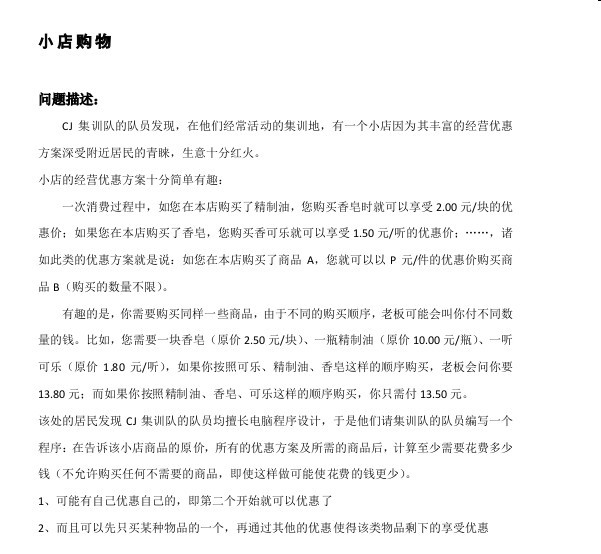Position：

# Bzoj 2260 商店购物

## Description

Grant是一个个体户老板，他经营的小店因为其丰富的优惠方案深受附近居民的青睐，生意红火。小店的优惠方案十分简单有趣。Grant规定：在一次消费过程中，如果您在本店购买了精制油的话，您购买香皂时就可以享受2.00元/块的优惠价；如果您在本店购买了香皂的话，您购买可乐时就可以享受1.50元/听的优惠价……诸如此类的优惠方案就是说：如果您在本店购买了商品A的话，您就可以以P元/件的优惠价格购买商品B（购买的数量不限）。有趣的是，你需要购买同样一些商品，由于不同的购买顺序，Grant老板可能会叫你付不同数量的钱。比如你需要一块香皂（原价2.50元）、一瓶精制油（原价10.00元）、一听可乐(原价1.80元)，如果你按照可乐，精制油，香皂这样的顺序购买的话，Grant老板会问你要13.80元；而如果你按照精制油，香皂，可乐这样的顺序购买的话，您只需付13.50元。

4
10.00 1
1.80 1
3.00 0
2.50 2
2
1 4 2.00
4 2 1.50

15.50

# Bzoj 4349 最小树形图

## Description

小C现在正要攻打科学馆腹地------计算机第三机房。而信息组的同学们已经建好了一座座堡垒，准备迎战。小C作为一种高度智慧的可怕生物，早已对同学们的信息了如指掌。

4
10.00 1
1.80 1
3.00 0
2.50 2
2
1 4 2.00
4 2 1.50

15.50

# CJ-test# Solution

## Notice:

1. 如果一个物品要买的数量为0，将其除去(为了算法的正常实行，可能图就不连通了)
2. Combine时注意新权值减去的是之前标号的s[x]
3. 注意自环的情况，见第一个break

# Code

// <shopping.cpp> - Wed Sep 14 08:15:57 2016
// This file is made by YJinpeng，created by XuYike's black technology automatically.
// Copyright (C) 2016 ChangJun High School, Inc.
// I don't know what this program is.

#include <iostream>
#include <vector>
#include <algorithm>
#include <cstring>
#include <cstdio>
#include <cstdlib>
#define MOD 1000000007
using namespace std;
typedef long long LL;
const int MAXN=100010;
const int MAXM=100010;
inline int max(int &x,int &y) {return x>y?x:y;}
inline int min(int &x,int &y) {return x<y?x:y;}
inline int gi() {
register int w=0,q=0;register char ch=getchar();
while((ch<'0'||ch>'9')&&ch!='-')ch=getchar();
if(ch=='-')q=1,ch=getchar();
while(ch>='0'&&ch<='9')w=w*10+ch-'0',ch=getchar();
return q?-w:w;
}
#define IN inline
#define RG register
struct Directed_MST{
static const int N=110,M=N*N;
static const double INF=1e9;
int m,n,root;
double w[M],s[N],ans,c[N];
int u[M],v[M],pre[N],id[N],k[N],f[N];
void Combine(){
for(int i=1;i<=m;i++){
int gg=v[i];u[i]=id[u[i]];v[i]=id[v[i]];
if(u[i]!=v[i])w[i]-=s[gg];//gg is important
}
}
void ZLEdmonds(){
while(true){
for(int i=1;i<=n;i++)s[i]=INF;
for(int i=1;i<=m;i++)
if(w[i]<s[v[i]]&&u[i]!=v[i])s[v[i]]=w[i],pre[v[i]]=u[i];
for(int i=1;i<=n;i++) {
if(i == root) continue;
if(s[i] == INF)    break;
}
int cnt=1;
memset(id,-1,sizeof(id));
memset(f,0,sizeof(f));
s[root]=0;
for(int i=1;i<=n;i++){
ans+=s[i];int x=i;
while(f[x]!=i&&x!=root)
f[x]=i,x=pre[x];
if(x!=root&&id[x]==-1){
for(int o=pre[x];o!=x;o=pre[o])
id[o]=cnt;
id[x]=cnt++;
}
}
if(cnt==1)break;
for(int i=1;i<=n;i++)if(id[i]==-1)id[i]=cnt++;
Combine();n=cnt-1;root=id[root];
}
printf("%.2lf",ans);
}
void Work(){
int cnt=gi();n=0;memset(id,0,sizeof(id));
for(int i=1;i<=cnt;i++){
scanf("%lf",&c[++n]);k[n]=gi()-1;
if(k[n]<0){n--;continue;}id[i]=n;
}
m=ans=0;n++;root=n;int mm=gi();
for(int i=1;i<n;i++)u[++m]=n,v[m]=i,w[m]=c[i];
for(int i=1;i<=mm;i++){
int x=gi(),y=gi();double p;scanf("%lf",&p);
if(!(id[x]&&id[y]))continue;
u[++m]=id[x];v[m]=id[y];w[m]=p;c[id[y]]=min(c[id[y]],p);
}
for(int i=1;i<n;i++)ans+=k[i]*c[i];
ZLEdmonds();
}
}ZL;
int main()
{
freopen("shopping.in","r",stdin);
freopen("shopping.out","w",stdout);
ZL.Work();
return 0;
}


posted @ 2016-09-30 21:58  _Mashiro  阅读(540)  评论(0编辑  收藏  举报